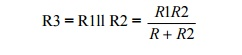Home | | Linear Integrated Circuits | IC723 as a LOW voltage LOW current

# IC723 as a LOW voltage LOW current

R1 & R2 from a potential divider between Vref& Gnd.

IC723 as a LOW voltage LOW current :

R1 & R2 from a potential divider between Vref& Gnd.

·           The Voltage across R2 is connected to the Non – inverting terminal of the regulator IC·           Gain of the internal error amplifier is largeTherefore the Vo is connected to the Inverting terminal through R3 & RSC must also be equal to Vnon-invR1 & R2 can be in the range of 1 KΩ to 10KΩ & value of R3 is given by·           Rsc (current sensing resistor) is connected between Cs & CL. The voltage drop across Rsc is proportional to the IL.

·           This resistor supplies the output voltage in the range of 2 to 7 volts, but the load current can be higher than 150mA.

·           The current sourcing capacity is increased by including a transistor Q in the circuitStudy Material, Lecturing Notes, Assignment, Reference, Wiki description explanation, brief detail
Linear Integrated Ciruits : Application of ICs : IC723 as a LOW voltage LOW current |